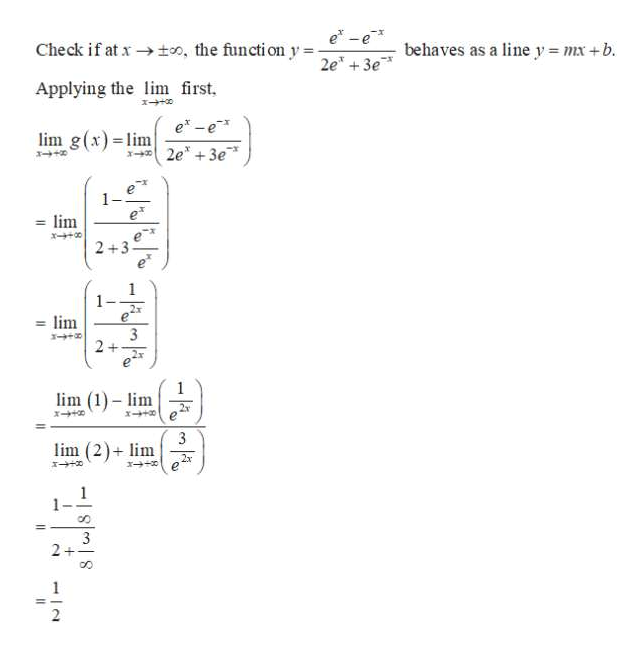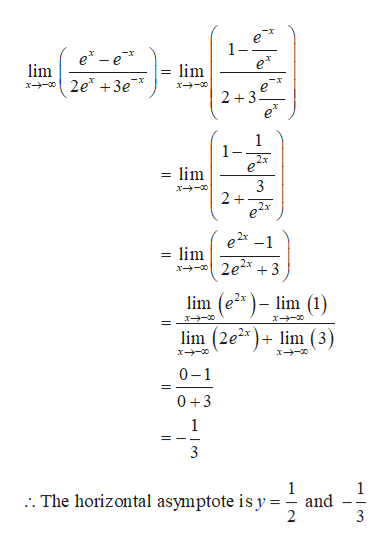# Find all asymptotes of g, where g(x) = (e^x-e^-x)/(2e^x+3e^-x)(use limits in your solution)

Question
2 views

Find all asymptotes of g, where g(x) = (e^x-e^-x)/(2e^x+3e^-x)(use limits in your solution)

check_circle

Step 1

The given function is,

Step 2

The horizontal asymptote is obtained as follows.help_outlineImage Transcriptionclosee* -e* Check if at x → too, the functi on y= behaves as a line y = mx +b. 2e" + 3e* Applying the lim first, e* -e* lim g(x) = lim x 2e* +3e* 1- = lim 2 +3 1- 2x = lim 3 2x lim (1) - lim 2x 3 lim (2)+ lim 2x 8. 3 fullscreen
Step 3

Also by the other way substitution of limit x tends to –in...help_outlineImage Transcriptionclosee* -e* e* lim lim x- 2+3e* e* 2e* + 3e* 1- = lim 3 2+ 2x 2x = lim x→- 2e2x +3 lim (e²* ) – lim (1) lim (2e2* )+ lim (3) 0-1 0 +3 3 .. The horizontal asymptote is y and 3 || fullscreen

### Want to see the full answer?

See Solution

#### Want to see this answer and more?

Solutions are written by subject experts who are available 24/7. Questions are typically answered within 1 hour.*

See Solution
*Response times may vary by subject and question.
Tagged in

### Functions